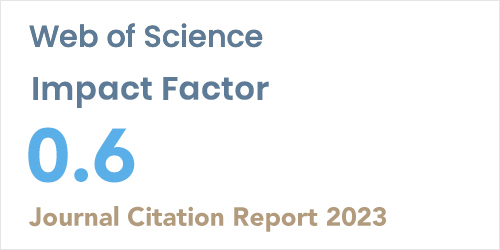ISSN: 1304-7191 | E-ISSN: 1304-7205
Volume: 41 Issue: 5 Year: 2023Novel analytical and approximate-analytical methods for solving the nonlinear fractional smoking mathematical model
1Department of Mathematics, Kafkas University, Kars, Türkiye
2Department of Mathematics and Statistics, Washington State University. Pullman, WA, USA
3Department of Mathematics,, Kyrgyz-Turkish Manas University,
Sigma J Eng Nat Sci - DOI: 10.14744/sigma.2021.00073

#### Abstract

Smoking is globally a challenging issue that causes many fatal health problems. In this paper, a nonlinear fractional smoking mathematical model is proposed in the context of a modified form of the Caputo fractional-order derivative. The analytical and approximate-analytical solutions are obtained for the proposed mathematical model via the fractional differential transform method (FDTM) and Laplace Adomian decomposition method (LADM). The obtained solution is provided as a rapidly convergent series. Simulation results are provided in this paper to compare the obtained solutions by FDTM, LADM, Runge Kutta (RK) method, and reduced differential transforms method (RDTM) with the exact solution of the proposed problem. By comparing both FDTM and LADM solutions, the FDTM solution is closer to the exact solution than the LADM solution. All obtained solutions have been analyzed and compared graphically to validate the effiency and applicability of all results.